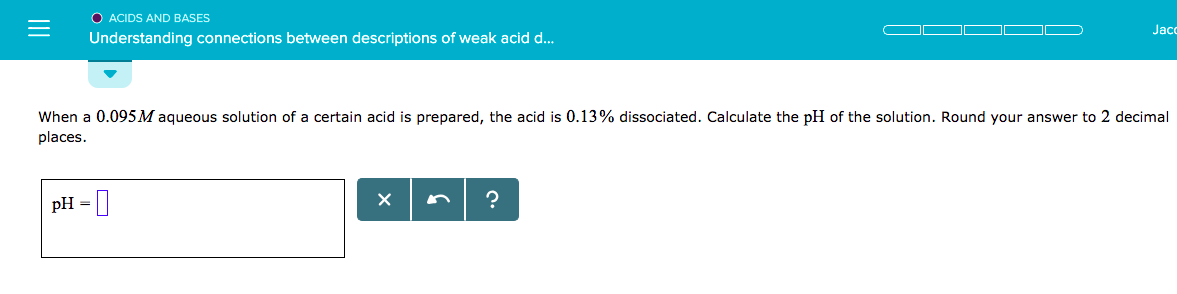# O ACIDS AND BASESUnderstanding connections between descriptions of weak acid d...Jaccwhen a 0.095M aqueous solution of a certain acid is prepared, the acid is 0.13% dissociated. Calculate the pH of the solution. Round your answer to 2 decimalplacespH-

Question
2 views

Acids & Bases: Understanding Connections Between Descriptions of Weak Acid Dissociations

When a 0.095M aqueous solution of a certain acid is prepared, the acid is 0.13% dissociated. Calculate the pH of the solution. Round your answer to 2 decimal places.

*PHOTO INCLUDED BELOWhelp_outlineImage TranscriptioncloseO ACIDS AND BASES Understanding connections between descriptions of weak acid d... Jacc when a 0.095M aqueous solution of a certain acid is prepared, the acid is 0.13% dissociated. Calculate the pH of the solution. Round your answer to 2 decimal places pH- fullscreen
check_circle

Step 1

The acid can be represented as HA. Acid dissociation is given. Here, one mole of acid dissociate to form one mole of H+ and one mole of A-.

Step 2

Initial concentration of the acid is 0.095 M. Here 0.13% of this acid gets dissociated. Amount of acid dissociated is calculated. Amount of acid dissociated and the concentration of H+ ion in the aqueous solution is same.

Step 3

pH of the solution can be determined from the concentration of H+ ion in...

### Want to see the full answer?

See Solution

#### Want to see this answer and more?

Solutions are written by subject experts who are available 24/7. Questions are typically answered within 1 hour.*

See Solution
*Response times may vary by subject and question.
Tagged in

### Properties of liquid